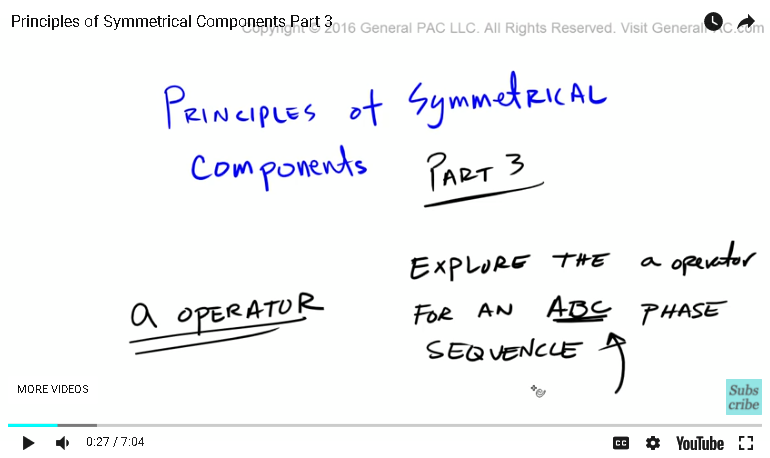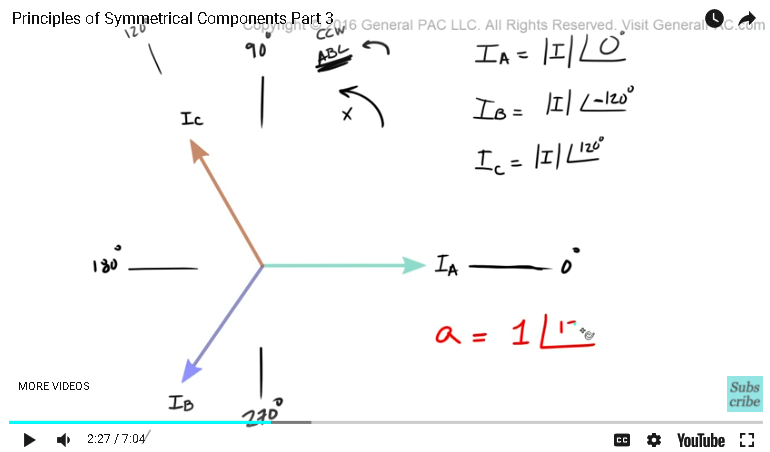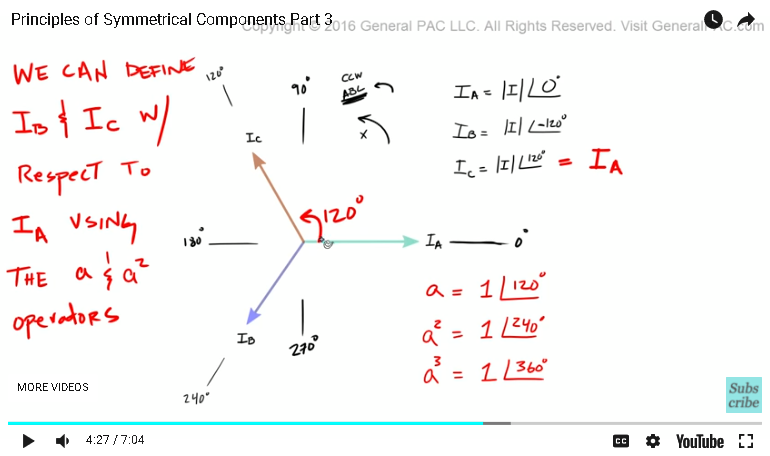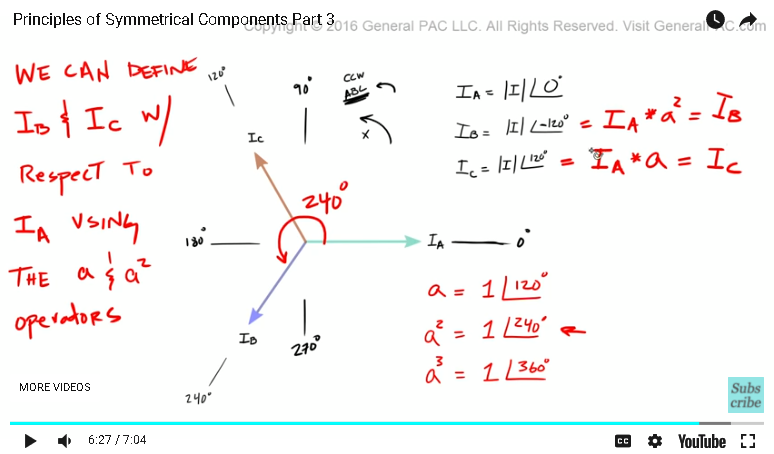Resources Section

Web Application for Symmetrical Componets: http://personal.strath.ac.uk/steven.m.blair/seq/

Do you have a question? Click on the "Questions & Answers forum" and ask away!

This video was brought to you by GeneralPAC.com, making power systems Intuitive, Open and Free for Everyone, Everywhere. Consider subscribing and supporting through patreon.com/GeneralPAC. This is a mechanism for you to support us financially so we can continue making high quality power system video tutorials. Our corporate sponsor for this topic is AllumiaX.com from Seattle, Washington. Contact them for industrial and commercial power system studies.

Principles of symmetrical components, part three. In this part we're going to explore this term called the A operator. Now if you already know what this is and how this works, you can safely skip this part. But if you really want to understand conceptually how this A operator works, then this is a great video tutorial for you.

So again, we're going to explore the A operator for an ABC phase sequence. It's really important to note that we're going to be working with an ABC phase sequence. So let's say we have a balanced system, again that was an ABC phase sequence. And let's just call this line here, we'll call this line our reference line, which is at zero degrees. And then, just imagine that this right here, this vertical line here, this was at 90 degrees with respect to this reference line. And then this right here was at 180 degrees, this right here, 270 degrees. So if you were to evaluate IA, we know that IA equals sum magnitude I. And it's in line with the reference line, which is pretty straightforward.Now if we were to evaluate IC, now this has 90 degrees, right? So IC, we're going to assume that IC was at 120 degrees. So IC equals sum magnitude I, right? Which is the same magnitude as IA, but now it's at 120 degrees with respect to the reference line. This is the reference line, so we're going 120 degrees in this direction and we get IC. So IC is equal to that.

Now, IB, we're going to say that it's at about 240 degrees, which means that IB is equal to sum magnitude I, but instead of going 240 degrees in this direction, we're going to go in the opposite direction 120 degrees. So we're going in the opposite direction 120 degrees. And we get IB.

So let's back up and let me put these definitions right here. Okay, so there we have it. So we're going to define the A operator as the number one, just the number one, and it's going to be at 120 degrees. Just the number one at 120 degrees. And then we're going to define the A superscript two operator and we're going to say the A super script two, is equal to one at 240 degrees.We're going to go one step further and we're going to define the A super script three operator, just to be clear, and we're going to define that as one at 36 degrees.

Now it's important to note and don't get confused by this, but this right here is A super script two, and this right here is A super script three. They're not A squared and they're not A cubed. The super script two and three is just for indication purposes.

So the beauty of this A operator is this. Here's what we can do with it and I'm going to write this down here. So we can define IB and IC with respect to IA using the A and A super script operators. So we can define IB and IC with respect to IA using these operators and that's the purpose of them. But that's not why we're watching this video. We want to get a very good visual understanding of how these A operators work.

Now check this out. We're going to start with IC first. So we know that IC is sum magnitude I at 120 degrees. So we're going 120 degrees like that and we get IC. So what if I said that IC, I'm going to define IC as just IA, right? So IA here. Now the question is what do I have to do to IA to make it overlap IC? Well, we know that IA has the same magnitude as IC, so we don't have to worry about the magnitudes. The only thing we have to worry about is the phase displacement between IA and IC. We know that the phase displacement is 120 degrees, right, so all we would need to do is take IA and then rotate it 120 degrees and we'll get IC. So that's exactly what we're going to do.We're going to take IA and then we're going to mathematically multiply it with just the A operator, right? Because we defined the A operator as one at 120 degrees, and what that'll do, is that'll shift IA 120 degrees in the counter clockwise direction. And if we do that, we get IC. Very, very simple, right? And then the same thing with IB. So IB is a little bit more trickier though. Again, the question is how do we represent IB with respect to IA? And the answer to that question is, well, IB and IA have the same magnitude, the only thing that's different is the phase displacement. And we'll take care of the phase displacement with this A operators. So if I take IA and I rotate it 240 degrees in the counter clockwise direction, then I know that I can overlap IB completely with just IA.

So that's exactly what we're going to do. We're going to take IB is equal to that. So we're going to take AI and then mathematically multiply it by a super script two and so what we're saying is we're going to take IA and then we're going to shift IA by 240 degrees. So we're going to take IA, shift it by 240 degrees and when we do that, we should get IB. Pretty simple, huh? Pretty simple.

All right. So, now, let's talk about this IA. IA is already at zero degrees, right? Technically we don't need to shift it, so we could just leave IA as that. So again, what we're doing with this A operator is that we are defining, right? IB and IC. So we're defining IB and IC with respect to IA, using the A operators. So this A operator's doing nothing but shifting phasors in a particular direction by a particular degree.Greetings from the GeneralPAC Team!

We make high-quality Power Systems Video Tutorials on complex topics that are free and open to everyone!  Thank you so much for supporting us through Patreon so can continue doing good and valuable work.

What is Patreon and why do we use it?

Patreon is a fantastic portal that allows our fans and community to make monthly contribution (like Netflix subscription) so we can continue creating high-quality power systems video tutorials. In return, you get access to incredible perks like voting on future topics, getting your questions answered, access to VIP Q/A webinars with the creators of GeneralPAC, and much more! We THANK YOU for supporting us

Why do we need your support?

An incredible amount of time and effort is needed to develop high-quality video tutorials. Each video (Part 1 for example) takes approximately 10 hours to complete which includes learning the concept ourselves, brainstorming creative ways to teach and explain the concepts, writing the script, audio recording, video recording, and editing. It's no wonder why Hundreds-of-Thousands of people have watched, liked, subscribed, and left positive comments on Youtube channel. Your support truly makes all the difference.